Most Affordable JEE | NEET | 8,9,10 Preparation by Kota's Top IITian Doctor Faculties

# NCERT Solutions for Class 12 Chemistry Chapter 5 Surface Chemistry PDF - eSaral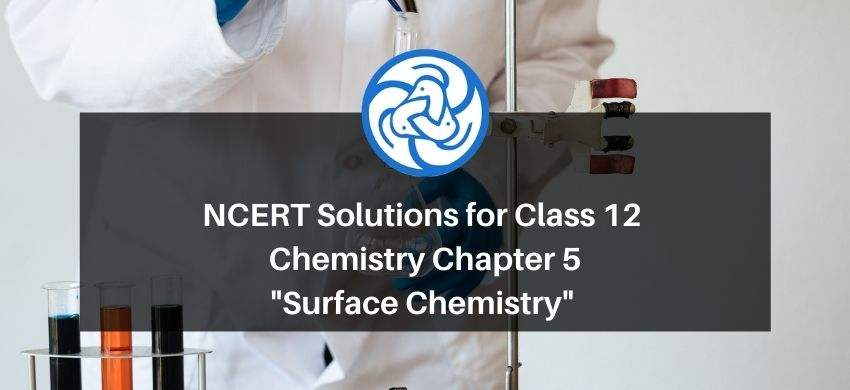# NCERT Solutions for Class 12 Chemistry Chapter 5 Surface Chemistry PDF

Hey, are you a class 12 student and looking for ways to download NCERT Solutions for Class 12 Chemistry Chapter 5 Surface Chemistry PDF? If yes. Then read this post till the end.

In this article, we have listed NCERT Solutions for Class 12 Chemistry Chapter 5 Surface Chemistry in PDF that are prepared by Kota’s top IITian Faculties by keeping Simplicity in mind.

If you want to learn and understand class 12 Chemistry Chapter 5 "Surface Chemistry" in an easy way then you can use these solutions PDF.

NCERT Solutions helps students to Practice important concepts of subjects easily. Class 12 Chemistry solutions provide detailed explanations of all the NCERT questions that students can use to clear their doubts instantly.

If you want to score high in your class 12 Chemistry Exam then it is very important for you to have a good knowledge of all the important topics, so to learn and practice those topics you can use eSaral NCERT Solutions.

So, without wasting more time Let’s start.

### Download NCERT Solutions for Class 12 Chemistry Chapter 5 Surface Chemistry PDF

Question 1: Write any two characteristics of Chemisorption.

Solution. 1. Chemisorption is highly specific in nature. It occurs only if there is a possibility of chemical bonding between the adsorbent and the adsorbate.

2. Like physisorption, chemisorption also increases with an increase in the surface area of the adsorbent.

Question 2: Why does physisorption decrease with the increase of temperature?

Solution. Physisorption is exothermic in nature. Therefore, in accordance with Le-Chateliere's principle, it decreases with an increase in temperature. This means that physisorption occurs more readily at a lower temperature.

Question 3: Why are powdered substances more effective adsorbents than their crystalline forms?

Solution. Powdered substances are more effective adsorbents than their crystalline forms because when a substance is powdered, its surface area increases and physisorption is directly proportional to the surface area of the adsorbent.

Question 4: Why is it necessary to remove CO when ammonia is obtained by Haber's process?

Solution. It is important to remove $\mathrm{CO}$ in the synthesis of ammonia as $\mathrm{CO}$ adversely affects the activity of the iron catalyst, used in Haber's process.

Question 5: Why is the ester hydrolysis slow in the beginning and becomes faster after sometime?

Solution. Ester hydrolysis can be represented as:

Ester + Water $\longrightarrow$ Acid $+$ Alcohol

The acid produced in the reaction acts as a catalyst and makes the reaction faster. Substances that act as catalysts in the same reaction in which they are obtained as products are known as autocatalysts.

Question 6: What is the role of desorption in the process of catalysis?

Solution. The role of desorption in the process of catalysis is to make the surface of the solid catalyst free for the fresh adsorption of the reactants on the surface.

Question 7: What modification can you suggest in the Hardy-Schulze law?

Solution. Hardy-Schulze law states that 'the greater the valence of the flocculating ion added, the greater is its power to cause precipitation.

This law takes into consideration only the charge carried by an ion, not its size. The smaller the size of an ion, the more will be its polarising power. Thus, Hardy-Schulze law can be modified in terms of the polarising power of the flocculating ion. Thus, the modified Hardy-Schulze law can be stated as 'the greater the polarising power of the flocculating ion added, the greater is its power to cause precipitation.

Question 8: Why is it essential to wash the precipitate with water before estimating it quantitatively?

Solution. When a substance gets precipitated, some ions that combine to form the precipitate get adsorbed on the surface of the precipitate. Therefore, it becomes important to wash the precipitate before estimating it quantitatively in order to remove these adsorbed ions or other such impurities.

Question 1: Distinguish between the meaning of the terms adsorption and absorption.

Give one example of each.

Solution. Adsorption is a surface phenomenon of accumulation of molecules of a substance at the surface rather than in the bulk of a solid or liquid. The substance that gets adsorbed is called the 'adsorbate' and the substance on whose surface the adsorption takes place is called the 'adsorbent'. Here, the concentration of the adsorbate on the surface of the adsorbent increases. In adsorption, the substance gets concentrated at the surface only. It does not penetrate through the surface to the bulk of the solid or liquid. For example, when we dip a chalk stick into an ink solution, only its surface becomes coloured. If we break the chalk stick, it will be found to be white from inside

On the other hand, the process of absorption is a bulk phenomenon. In absorption, the substance gets uniformly distributed throughout the bulk of the solid or liquid.

Question 2: What is the difference between physisorption and chemisorption?

Solution.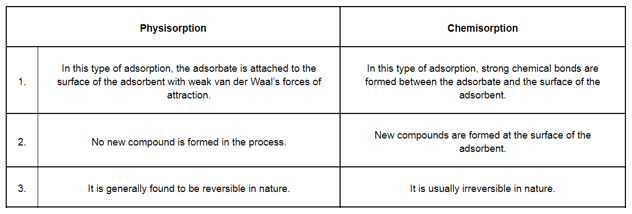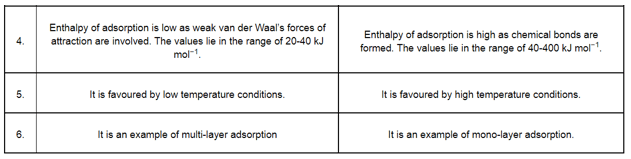Question 3: Give reason why a finely divided substance is more effective as an adsorbent.

Solution. Adsorption is a surface phenomenon. Therefore, adsorption is directly proportional to the surface area. A finely divided substance has a large surface area. Both physisorption and chemisorption increase with an increase in the surface area. Hence, a finely divided substance behaves as a good adsorbent.

Question 4: What are the factors which influence the adsorption of a gas on a solid?

Solution. There are various factors that affect the rate of adsorption of a gas on a solid surface.

(1) Nature of the gas:

Easily liquefiable gases such as $\mathrm{NH}_{3}, \mathrm{HCl}$ etc. are adsorbed to a great extent in comparison to gases such as $\mathrm{H}_{2}, \mathrm{O}_{2}$ etc. This is because Van der Waal's forces are stronger in easily liquefiable gases.

(2) Surface area of the solid

The greater the surface area of the adsorbent, the greater is the adsorption of a gas on the solid surface.

(3) Effect of pressure

Adsorption is a reversible process and is accompanied by a decrease in pressure. Therefore, adsorption increases with an increase in pressure.

(4) Effect of temperature

Adsorption is an exothermic process. Thus, in accordance with Le-Chatelier's principle, the magnitude of adsorption decreases with an increase in temperature.

Solution.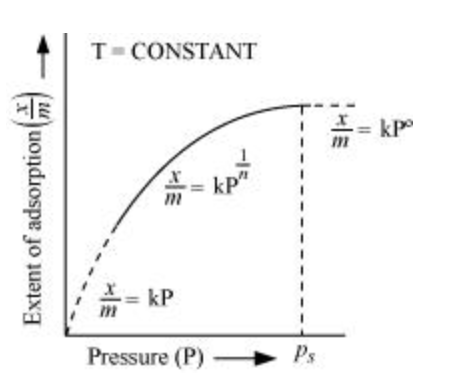The plot between the extent of adsorption $\left(\frac{x}{m}\right)$ against the pressure of gas $(P)$ at constant temperature ( $\mathrm{T}$ ) is called the adsorption isotherm.

Freundlich adsorption isotherm gives an empirical relationship between the quantity of gas adsorbed by the unit mass of solid adsorbent and pressure at a specific temperature.

From the given plot it is clear that at pressure $P_{\mathrm{S}}, \frac{x}{m}$ reaches the maximum valve. $P_{s}$ is called the saturation pressure. Three cases arise from the graph now.

Case I- At low pressure:

The plot is straight and sloping, indicating that the pressure in directly proportional to $\frac{x}{m}$ i.e., $\frac{x}{m} \alpha P$.

$\frac{x}{m}=k P$

( $k$ is a constant)

Case II- At high pressure:

When pressure exceeds the saturated pressure, $\frac{x}{m}$becomes independent of P values.

$\frac{x}{m} \alpha P^{\circ}$

$\frac{x}{m}=k P^{\circ}$

Case III- At intermediate pressure:

At intermediate pressure, $\frac{x}{m}$ depends on $P$ raised to the powers between 0 and $1 .$ This relationship is known as the Freundlich adsorption isotherm.

$\frac{x}{m} \alpha P^{\frac{1}{n}}$

$\frac{x}{m}=k P^{\frac{1}{n}} \quad n>1$

Now, taking log:

$\log \frac{x}{m}=\log k+\frac{1}{n} \log P$

On plotting the graph between $\log \left(\frac{x}{m}\right)$ and $\log P$, a straight line is obtained with the slope equal to $-$ and the intercept equal to log $\mathrm{k}$.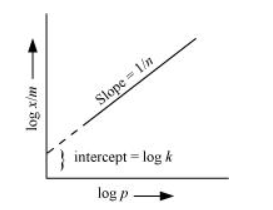Question 6: What do you understand by activation of adsorbent? How is it achieved?

(i) By increasing the surface area of the adsorbent. This can be done by breaking it into smaller pieces or powdering it.

(ii) Some specific treatments can also lead to the activation of the adsorbent. For example, wood charcoal is activated by heating it between $650 \mathrm{~K}$ and $1330 \mathrm{~K}$ in vacuum or air. It expels all the gases absorbed or adsorbed and thus, creates a space for adsorption of gases.

Question 7: What role does adsorption play in heterogeneous catalysis?

Solution. Heterogeneous catalysis:

A catalytic process in which the catalyst and the reactants are present in different phases is known as a heterogeneous catalysis. This heterogeneous catalytic action can be explained in terms of the adsorption theory. The mechanism of catalysis involves the following steps:

(i) Adsorption of reactant molecules on the catalyst surface.

(ii) Occurrence of a chemical reaction through the formation of an intermediate.

(iii) De-sorption of products from the catalyst surface

(iv) Diffusion of products away from the catalyst surface.

In this process, the reactants are usually present in the gaseous state and the catalyst is present in the solid state. Gaseous molecules are then adsorbed on the surface of the catalyst. As the concentration of reactants on the surface of the catalyst increases, the rate of reaction also increases. In such reactions, the products have very less affinity for the catalyst and are quickly desorbed, thereby making the surface free for other reactants.

Question 8: Why is adsorption always exothermic?

Solution. Adsorption is always exothermic. This statement can be explained in two ways.

(i) Adsorption leads to a decrease in the residual forces on the surface of the adsorbent. This causes a decrease in the surface energy of the adsorbent. Therefore, adsorption is always exothermic.

(ii) $\Delta H$ of adsorption is always negative. When a gas is adsorbed on a solid surface, its movement is restricted leading to a decrease in the entropy of the gas i.e., $\Delta S$ is negative. Now for a process to be spontaneous, $\Delta G$ should be negative.

$\therefore \Delta G=\Delta H-T \Delta S$

Since $\Delta S$ is negative, $\Delta H$ has to be negative to make $\Delta G$ negative. Hence, adsorption is always exothermic.

Question 9: How are the colloidal solutions classified on the basis of physical states of the dispersed phase and dispersion medium?

Solution. One criterion for classifying colloids is the physical state of the dispersed phase and dispersion medium. Depending upon the type of the dispersed phase and dispersion medium (solid, liquid, or gas), there can be eight types of colloidal systems.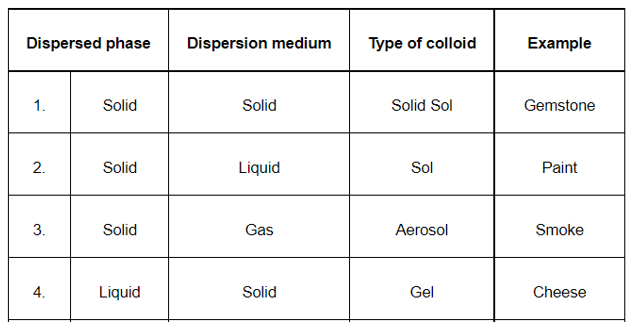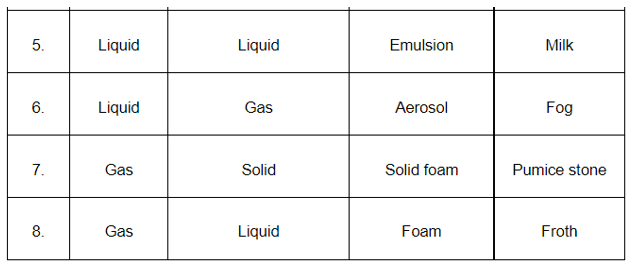Question 10: Discuss the effect of pressure and temperature on the adsorption of gases on solids.

Solution. Effect of pressure

Adsorption is a reversible process and is accompanied by a decrease in pressure. Therefore, adsorption increases with an increase in pressure.

Effect of temperature

Adsorption is an exothermic process. Thus, in accordance with Le-Chatelier’s principle, the magnitude of adsorption decreases with an increase in temperature.

Question 11: What are lyophilic and lyophobic sols? Give one example of each type. Why are hydrophobic sols easily coagulated?

Solution. (i) Lyophilic sols:

Colloidal sols that are formed by mixing substances such as gum, gelatin, starch, etc. with a suitable liquid (dispersion medium) are called lyophilic sols. These sols are reversible in nature i.e., if two constituents of the sol are separated by any means (such as evaporation), then the sol can be prepared again by simply mixing the dispersion medium with the dispersion phase and shaking the mixture.

(ii) Lyophobic sols:

When substances such as metals and their sulphides etc. are mixed with the dispersion medium, they do not form colloidal sols. Their colloidal sols can be prepared only by special methods. Such sols are called lyophobic sols. These sols are irreversible in nature. For example: sols of metals.

Now, the stability of hydrophilic sols depends on two things- the presence of a charge and the salvation of colloidal particles. On the other hand, the stability of hydrophobic sols is only because of the presence of a charge. Therefore, the latter are much less stable than the former. If the charge of hydrophobic sols is removed (by addition of electrolytes), then the particles present in them come closer and form aggregates, leading to precipitation.

Question 12: What is the difference between multimolecular and macromolecular colloids? Give one example of each. How are associated colloids different from these two types of colloids?

Solution. (i) In multi-molecular colloids, the colloidal particles are an aggregate of atoms or small molecules with a diameter of less than 1 nm. The molecules in the aggregate are held together by van der Waal’s forces of attraction. Examples of such colloids include gold sol and sulphur sol.

(ii) In macro-molecular colloids, the colloidal particles are large molecules having colloidal dimensions. These particles have a high molecular mass. When these particles are dissolved in a liquid, sol is obtained. For example: starch, nylon, cellulose, etc.

(iii) Certain substances tend to behave like normal electrolytes at lower concentrations. However, at higher concentrations, these substances behave as colloidal solutions due to the formation of aggregated particles. Such colloids are called aggregated colloids.

Question 13: What are enzymes? Write in brief the mechanism of enzyme catalysis.

Solution. Enzymes are basically protein molecules of high molecular masses. These form colloidal solutions when dissolved in water. These are complex, nitrogenous organic compounds produced by living plants and animals. Enzymes are also called 'biochemical catalysts'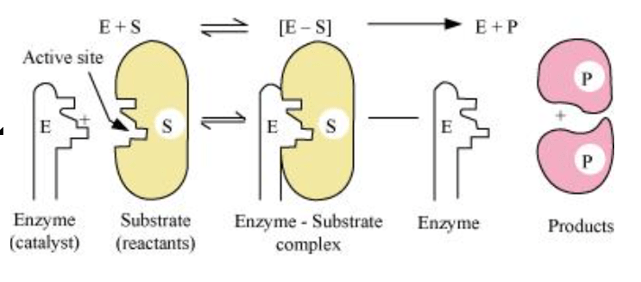Mechanism of enzyme catalysis:

On the surface of the enzymes, various cavities are present with characteristic shapes. These cavities possess active groups such as $-\mathrm{NH}_{2},-\mathrm{COOH}$, etc. The reactant molecules having a complementary shape fit into the cavities just like a key fits into a lock. This leads to the formation of an activated complex. This complex then decomposes to give the product.

Hence,

Step $1: \mathrm{E}+\mathrm{S} \rightarrow \mathrm{ES}^{+}$

(Activated complex)

Step 2: $\mathrm{ES}^{+} \rightarrow \mathrm{E}+\mathrm{P}$

Question 14: How are colloids classified on the basis of

(i) Physical states of components

(ii) Nature of dispersion medium and

(iii) Interaction between dispersed phase and dispersion medium?

Solution. Colloids can be classified on various bases:

(i) On the basis of the physical state of the components (by components we mean the dispersed phase and dispersion medium). Depending on whether the components are solids, liquids, or gases, we can have eight types of colloids.

(ii) On the basis of the dispersion medium, sols can be divided as: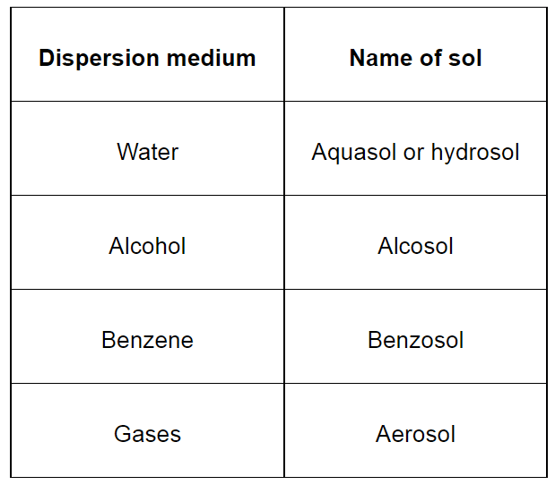(iii) On the basis of the nature of the interaction between the dispersed phase and dispersion medium, the colloids can be classified as lyophilic (solvent attracting) and lyophobic (solvent repelling).

Question 15: Explain what is observed

(i) When a beam of light is passed through a colloidal sol.

(ii) An electrolyte, NaCl is added to hydrated ferric oxide sol.

(iii) Electric current is passed through a colloidal sol?

Solution. (i) When a beam of light is passed through a colloidal solution, then scattering of light is observed. This is known as the Tyndall effect. This scattering of light illuminates the path of the beam in the colloidal solution.

(ii) When NaCl is added to ferric oxide sol, it dissociates to give Na+ and Cl-¬ ions. Particles of ferric oxide sol are positively charged. Thus, they get coagulated in the presence of negatively charged Cl- ions.

(iii) The colloidal particles are charged and carry either a positive or negative charge. The dispersion medium carries an equal and opposite charge. This makes the whole system neutral. Under the influence of an electric current, the colloidal particles move towards the oppositely charged electrode. When they come in contact with the electrode, they lose their charge and coagulate.

Question 16: What are emulsions? What are their different types? Give example of each type.

Solution. The colloidal solution in which both the dispersed phase and dispersion medium are liquids is called an emulsion.

There are two types of emulsions:

(a) Oil in water type:

Here, oil is the dispersed phase while water is the dispersion medium. For example: milk, vanishing cream, etc.

(b) Water in oil type:

Here, water is the dispersed phase while oil is the dispersion medium. For example: cold cream, butter, etc.

Question 17: What is demulsification? Name two demulsifiers.

Solution. The process of decomposition of an emulsion into its constituent liquids is called demulsification. Examples of demulsifiers are surfactants, ethylene oxide, etc.

Question 18: Action of soap is due to emulsification and micelle formation. Comment.

Solution. The cleansing action of soap is due to emulsification and micelle formation. Soaps are basically sodium and potassium salts of long chain fatty acids, $\mathrm{R}-\mathrm{COO}^{-} \mathrm{Na}^{+}$. The end of the molecule to which the sodium is attached is polar in nature, while the alkyl-end is non-polar. Thus, a soap molecule contains a hydrophilic (polar) and a hydrophobic (non-polar) part.

When soap is added to water containing dirt, the soap molecules surround the dirt particles in such a manner that their hydrophobic parts get attached to the dirt molecule and the hydrophilic parts point away from the dirt molecule. This is known as micelle formation. Thus, we can say that the polar group dissolves in water while the non-polar group dissolves in the dirt particle. Now, as these micelles are negatively charged, they do not coalesce and a stable emulsion is formed.

Question 19: Give four examples of heterogeneous catalysis.

Solution. (i) Oxidation of sulphur dioxide to form sulphur trioxide. In this reaction, Pt acts as a catalyst.

$2 \mathrm{SO}_{2(g)} \stackrel{\mathrm{Pt}_{(g)}}{\longrightarrow} 2 \mathrm{SO}_{3(g)}$

(ii) Formation of ammonia by the combination of dinitrogen and dihydrogen in the presence of finely divided iron.

$\mathrm{N}_{2(g)}+3 \mathrm{H}_{2(g)} \stackrel{\mathrm{Fe}_{(g)}}{\longrightarrow} 2 \mathrm{NH}_{3(g)}$

This process is called the Haber's process.

(iii) Oswald's process: Oxidation of ammonia to nitric oxide in the presence of platinum.

$4 \mathrm{NH}_{3(g)}+5 \mathrm{O}_{2(g)} \stackrel{\mathrm{P}_{(\mathrm{g})}}{\longrightarrow} 4 \mathrm{NO}_{(g)}+6 \mathrm{H}_{2} \mathrm{O}_{(g)}$

(iv) Hydrogenation of vegetable oils in the presence of Ni.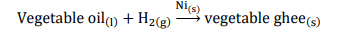Question 20: What do you mean by activity and selectivity of catalysts?

Solution. (a) Activity of a catalyst:

The activity of a catalyst is its ability to increase the rate of a particular reaction. Chemisorption is the main factor in deciding the activity of a catalyst. The adsorption of reactants on the catalyst surface should be neither too strong nor too weak. It should just be strong enough to make the catalyst active.

(b) Selectivity of the catalyst:

The ability of the catalyst to direct a reaction to yield a particular product is referred to as the selectivity of the catalyst. For example, by using different catalysts, we can get different products for the reaction between $\mathrm{H}_{2}$ and $\mathrm{CO}$.

(i) $\mathrm{CO}_{(g)}+3 \mathrm{H}_{2(g)} \stackrel{\mathrm{Ni}}{\longrightarrow} \mathrm{CH}_{4(g)}+\mathrm{H}_{2} \mathrm{O}_{(g)}$

(ii) $\mathrm{CO}_{(g)}+2 \mathrm{H}_{2(g)} \stackrel{\mathrm{CuZnO}-\mathrm{CrO}_{3}}{\longrightarrow} \mathrm{CH}_{3} \mathrm{OH}_{(g)}$

(iii) $\mathrm{CO}_{(g)}+\mathrm{H}_{2(g)} \stackrel{\mathrm{Cu}}{\longrightarrow} \mathrm{HCHO}_{(g)}$

Question 21: Describe some features of catalysis by zeolites.

Solution. Zeolites are alumino-silicates that are micro-porous in nature. Zeolites have a honeycomb-like structure, which makes them shape-selective catalysts. They have an extended 3D-network of silicates in which some silicon atoms are replaced by aluminium atoms, giving them an $\mathrm{Al}-\mathrm{O}-\mathrm{Si}$ framework. The reactions taking place in zeolites are very sensitive to the pores and cavity size of the zeolites. Zeolites are commonly used in the petrochemical industry.

Question 22: What is shape selective catalysis?

Solution. A catalytic reaction which depends upon the pore structure of the catalyst and on the size of the reactant and the product molecules is called shape-selective catalysis. For example, catalysis by zeolites is a shape-selective catalysis. The pore size present in the zeolites ranges from 260-740 pm. Thus, molecules having a pore size more than this cannot enter the zeolite and undergo the reaction.

Question 23: Explain the following terms:

(i) Electrophoresis

(ii) Coagulation

(iii) Dialysis

(iv) Tyndall effect.

Solution. (i) Electrophoresis:

The movement of colloidal particles under the influence of an applied electric field is known as electrophoresis. Positively charged particles move to the cathode, while negatively charged particles move towards the anode. As the particles reach oppositely charged electrodes, they become neutral and get coagulated.

(ii) Coagulation:

The process of settling down of colloidal particles i.e., conversion of a colloid into a precipitate is called coagulation.

(iii) Dialysis

The process of removing a dissolved substance from a colloidal solution by the means of diffusion through a membrane is known as dialysis. This process is based on the principle that ions and small molecules can pass through animal membranes unlike colloidal particles.

(iv) Tyndall effect:

When a beam of light is allowed to pass through a colloidal solution, it becomes visible like a column of light. This is known as the Tyndall effect. This phenomenon takes place as particles of colloidal dimensions scatter light in all directions.

Question 24: Give four uses of emulsions.

Solution. (i) Cleansing action of soaps is based on the formation of emulsions.

(ii) Digestion of fats in intestines takes place by the process of emulsification.

(iii) Antiseptics and disinfectants when added to water form emulsions.

(iv) The process of emulsification is used to make medicines.

Question 25: What are micelles? Give an example of a micellers system.

Solution. Micelle formation is done by substances such as soaps and detergents when dissolved in water. The molecules of such substances contain a hydrophobic and a hydrophilic part. When present in water, these substances arrange themselves in spherical structures in such a manner that their hydrophobic parts are present towards the centre, while the hydrophilic parts are pointing towards the outside (as shown in the given figure). This is known as micelle formation.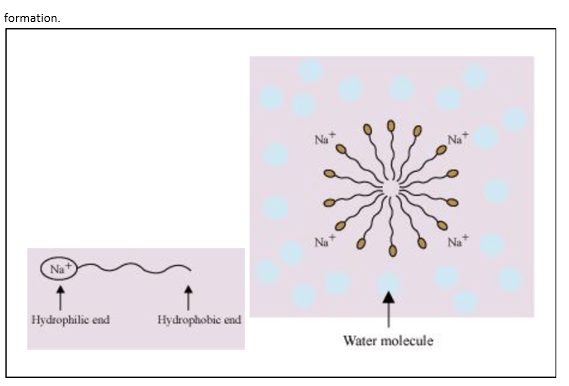Question 26: Explain the terms with suitable examples:

(i) Alcosol

(ii) Aerosol

(iii) Hydrosol

Solution. (i) Alcosol:

A colloidal solution having alcohol as the dispersion medium and a solid substance as the dispersed phase is called an alcosol.

For example: colloidal sol of cellulose nitrate in ethyl alcohol is an alcosol.

(ii) Aerosol:

A colloidal solution having a gas as the dispersion medium and a solid as the dispersed phase is called an aerosol.

For example: fog

(iii) Hydrosol

A colloidal solution having water as the dispersion medium and a solid as the dispersed phase is called a hydrosol.

For example: starch sol or gold sol

Question 27: Comment on the statement that "colloid is not a substance but a state of substance".

Solution. Common salt (a typical crystalloid in an aqueous medium) behaves as a colloid in a benzene medium. Hence, we can say that a colloidal substance does not represent a separate class of substances. When the size of the solute particle lies between 1 nm and 1000 nm, it behaves as a colloid.

Hence, we can say that colloid is not a substance but a state of the substance which is dependent on the size of the particle. A colloidal state is intermediate between a true solution and a suspension.